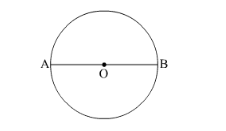# If the coordinates of one end of a diameter of a circle are (2, 3)Question:

If the coordinates of one end of a diameter of a circle are (2, 3) and the coordinates of its centre are (−2, 5), then the coordinates of the other end of the diameter are

(a) (−6, 7)

(b) $(6,-7)$

(c) $(6,7)$

(d) $(-6,-7)$                                                                [CBSE 2012]

Solution:Let O(−2, 5) be the centre of the given circle and A(2, 3) and B(xy) be the end points of a diameter of the circle.

Then, O is the mid-point of AB.

Using mid-point formula, we have

$\therefore \frac{2+x}{2}=-2$ and $\frac{3+y}{2}=5$

$\Rightarrow 2+x=-4$ and $3+y=10$

$\Rightarrow x=-6$ and $y=7$

Thus, the coordinates of the other end of the diameter are (−6, 7).

Hence, the correct answer is option A.